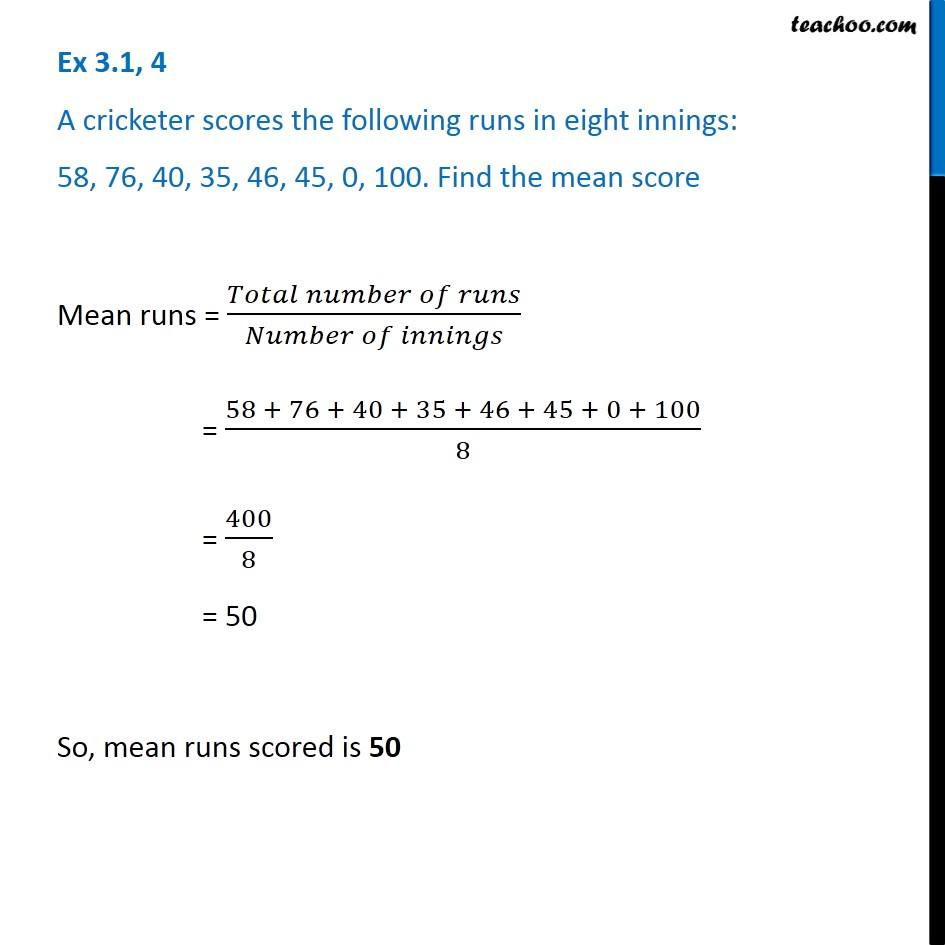Mean

Chapter 3 Class 7 Data Handling
Concept wiseIntroducing your new favourite teacher - Teachoo Black, at only ₹83 per month

### Transcript

Ex 3.1, 4 A cricketer scores the following runs in eight innings: 58, 76, 40, 35, 46, 45, 0, 100. Find the mean score Mean runs = (𝑇𝑜𝑡𝑎𝑙 𝑛𝑢𝑚𝑏𝑒𝑟 𝑜𝑓 𝑟𝑢𝑛𝑠)/(𝑁𝑢𝑚𝑏𝑒𝑟 𝑜𝑓 𝑖𝑛𝑛𝑖𝑛𝑔𝑠) = (58 + 76 + 40 + 35 + 46 + 45 + 0 + 100)/8 = 400/8 = 50 So, mean runs scored is 50